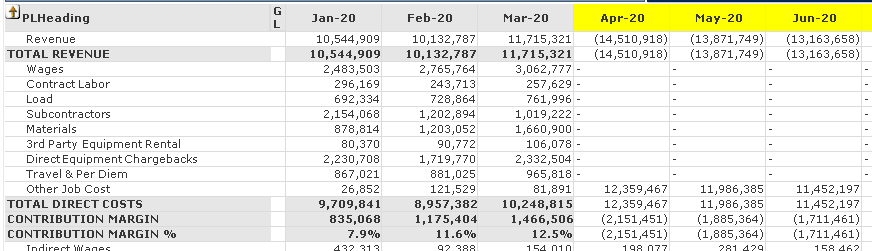# QlikView App Dev

Discussion Board for collaboration related to QlikView App Development.

Announcements
QlikWorld 2023, a live, in-person thrill ride. Save \$300 before February 6: REGISTER NOW!
cancel
Showing results for
Did you mean:Contributor

## Profit and Loss Script Issue

I need help regarding my Profit and Lost table expression that works on Actuals (Jan-20, Feb-20, Mar-20 columns), however the +/- sign is negative for Budget numbers (Apr-20, May-20, Jun-20 columns) and the Contribution Margin % wont calculate. My current selections are the Actuals (Jan-20, Feb-20, Mar-20 columns) in this example.SCRIPT:

vMonth=Max([Month Year])

=If(PLSign=1,
Num(if(Only({1}[Month Year])<=vMonth, sum({<[Month Year],Ledger_Type={'Actual'}>}-Amount), sum({<[Month Year],Ledger_Type={'Plan'}>}-Amount)),'#,##0;(#,##0)')
,If(PLLevel = 'p'
, Num(if(Only({1}[Month Year])<=vMonth, sum({<[Month Year],Ledger_Type={'Actual'}>}-Amount), sum({<[Month Year],Ledger_Type={'Plan'}>}-Amount))
/if(Only({1}[Month Year])<=vMonth, sum({<[Month Year],Ledger_Type={'Actual'}>}If(Object_Account>='400000' AND Object_Account<='499999',-Amount)), sum({<[Month Year],Ledger_Type={'Plan'}>}If(Object_Account>='400000' AND Object_Account<='499999',-Amount))),'#,##0.0%')
,If(Num(if(Only({1}[Month Year])<=vMonth, sum({<[Month Year],Ledger_Type={'Actual'}>}-Amount), sum({<[Month Year],Ledger_Type={'Plan'}>}-Amount)), '#,##0;(#,##0)')
, Num(if(Only({1}[Month Year])<=vMonth, sum({<[Month Year],Ledger_Type={'Actual'}>}Amount), sum({<[Month Year],Ledger_Type={'Plan'}>}Amount)), '#,##0;(#,##0)')
)
)
)

Other notes: the PLSign is to switch sign since the data base shows revenue as a negative. PLLevel with a P identifies the PL Group should be a “%”.

Labels (8)

• ### scripting help